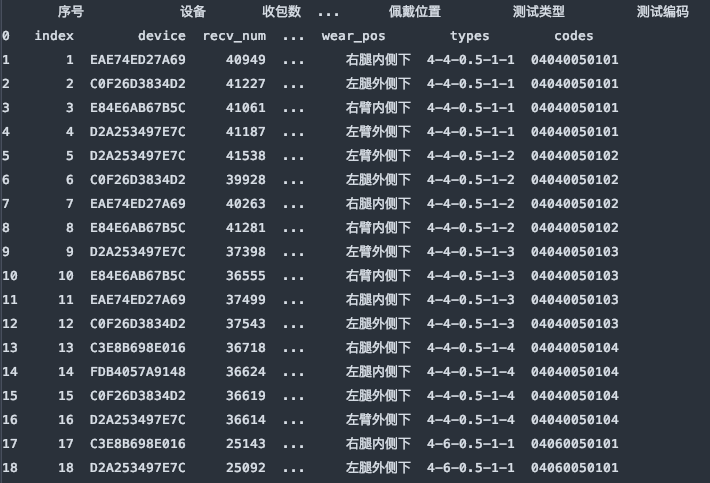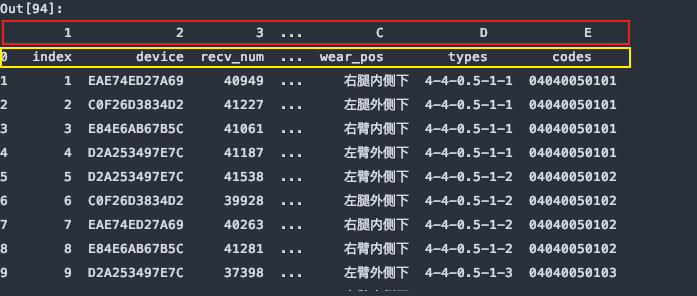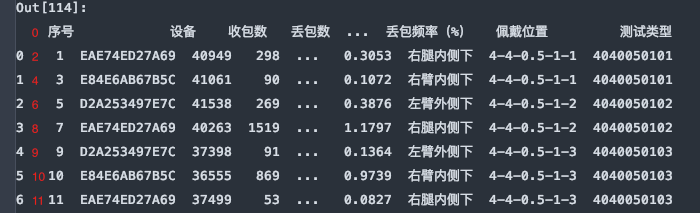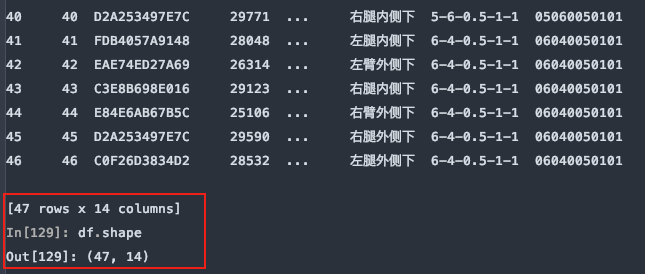# pandas 读取excel文件

### pandas 读取excel文件

• 三 示例
• 1. IO：路径
• 2. sheet_name：指定工作表名
• 4. names： 指定列名
• 5. index_col: 指定列索引
• 6. skiprows：跳过指定行数的数据
• 7. skipfooter：省略从尾部的行数据
• 8.dtype 指定某些列的数据类型

``````import pandas as pd

file_name = 'xxx.xlsx'
``````

1. io: excel路径 可以是文件路径， 类文件对象， 文件路径对象等。

2. sheet_name=0: 访问指定excel某张工作表。sheet_name可以是str, int, list 或 None类型， 默认值是0。

3. str类型 是直接指定工作表的名称

4. int类型 是指定从0开始的工作表的索引， 所以sheelt_name默认值是0，即第一个工作表。

5. list类型 是多个索引或工作表名构成的list，指定多个工作表。

6. None类型， 访问所有的工作表

7. sheet_name=0: 得到的是第1个sheet的DataFrame类型的数据

8. sheet_name=2: 得到的是第3个sheet的DataFrame类型的数据

9. sheet_name=‘Test1’: 得到的是名为’Test1’的sheet的DataFrame类型的数据

10. sheet_name=[0, 3, ‘Test5’]: 得到的是第1个，第4个和名为Test5 的工作表作为DataFrame类型的数据的字典。

12. name=None： 传入一列类数组类型的数据，用来作为数据的列名。`如果文件数据不包含标题行，要显式的指出header=None`

13. skiprows：int类型， 类列表类型或可调函数。 要跳过的行号(0索引)或文件开头要跳过的行数(int)。如果可调用，可调用函数将根据行索引进行计算，如果应该跳过行则返回True，否则返回False。一个有效的可调用参数的例子是lambda x: x in [0, 1, 2]。

14. skipfooter=0: int类型， 默认0。自下而上，从尾部指定跳过行数的数据。

15. usecols=None: 指定要使用的列，如果没有默认解析所有的列。

16. index_col=None: int或元素都是int的列表， 将某列的数据作为DataFrame的行标签，如果传递了一个列表，这些列将被组合成一个多索引，如果使用usecols选择的子集，index_col将基于该子集。

17. squeeze=False, 布尔值，默认False。 如果解析的数据只有一列，返回一个Series。

18. dtype=None: 指定某列的数据类型，可以使类型名或一个对应列名与类型的字典，例 `{‘A’: np.int64, ‘B’: str} `

19. nrows=None: int类型，默认None。 只解析指定行数的数据。

## 三 示例

如图是演示使用的excel文件，它包含5张工作表。### 1. IO：路径

举一个IO为文件对象的例子， 有些时候file文件路径的包含较复杂的中文字符串时，pandas 可能会解析文件路径失败，可以使用文件对象来解决。

``````file = 'xxxx.xlsx'
f = open(file, 'rb')

f.close()  # 没有使用with的话，记得要手动释放。

# ------------- with模式 -------------------
with open(file, 'rb') as f:
``````

### 2. sheet_name：指定工作表名

sheet_name=‘Sheet’， 指定解析名为"Sheet1"的工作表。返回一个DataFrame类型的数据。

``````df = pd.read_excel(file, sheet_name='Sheet1')
``````sheet_name=[0, 1, ‘Sheet1’], 对应的是解析文件的第1， 2张工作表和名为"Sheet1"的工作表。它返回的是一个有序字典。结构为{name：DataFrame}这种类型。

``````df_dict = pd.read_excel(file, sheet_name=[0,1,'Sheet1'])
``````sheet_name=None 会解析该文件中所有的工作表，返回一个同上的字典类型的数据。

``````df_dict = pd.read_excel(file, sheet_name=None)
````````````df_dict = pd.read_excel(file, sheet_name='Sheet1')
````````````df_dict = pd.read_excel(file, sheet_name='Sheet1', header=1)
````````````df_dict = pd.read_excel(file, sheet_name='Sheet1', header=None)
``````### 4. names： 指定列名

指定数据的列名，如果数据已经有列名了，会替换掉原有的列名。

``````df = pd.read_excel(file, sheet_name='Sheet1', names=list('123456789ABCDE'))
````````````df = pd.read_excel(file, sheet_name='Sheet1', names=list('123456789ABCDE'), header=None)
``````### 5. index_col: 指定列索引

``````df = pd.read_excel(file, sheet_name='Sheet1', header=1, index_col=0)
``````### 6. skiprows：跳过指定行数的数据

``````df = pd.read_excel(file, sheet_name='Sheet1', skiprows=0)
````````````df = pd.read_excel(file, sheet_name='Sheet1', skiprows=[1,3,5,7,9,])
````````````df = pd.read_excel(file, sheet_name='Sheet1', header=5)
````````````df = pd.read_excel(file, sheet_name='Sheet1', skiprows=5)
``````### 7. skipfooter：省略从尾部的行数据

原始的数据有47行，如下图所示：从尾部跳过5行：

``````df = pd.read_excel(file, sheet_name='Sheet1', skipfooter=5)
``````### 8.dtype 指定某些列的数据类型

示例数据中，测试编码数据是文本，而pandas在解析的时候自动转换成了int64类型，这样codes列的首位0就会消失，造成数据错误，如下图所示指定codes列的数据类型：

``````df = pd.read_excel(file, sheet_name='Sheet1', header=1, dtype={'codes': str})
``````来源：littleRpl

物联沃分享整理
物联沃-IOTWORD物联网 » pandas 读取excel文件